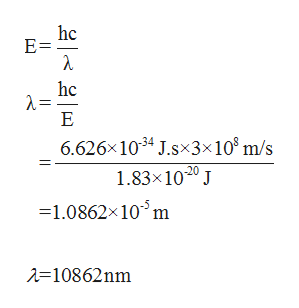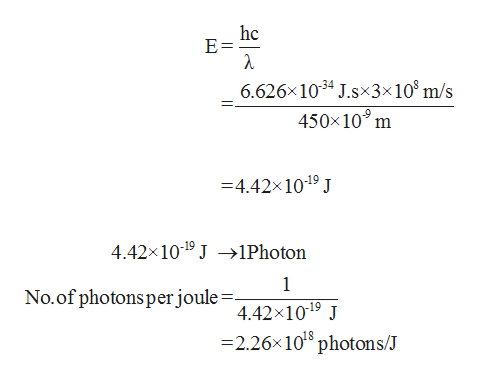# Calculate the wavelength (in nm) of light with energy 1.83×10−20 J per photon. For light of wavelength 450 nm, calculate the number of photons per joule.Enter your answer in scientific notation. Determine the binding energy (in eV) of a metal if the kinetic energy possessed by an ejected electron (using one of the photons with wavelength 450 nm) is 2.93×10−19 J.

Question
185 views

Calculate the wavelength (in nm) of light with energy 1.83×10−20 J per photon.

For light of wavelength 450 nm, calculate the number of photons per joule.

Determine the binding energy (in eV) of a metal if the kinetic energy possessed by an ejected electron (using one of the photons with wavelength 450 nm) is 2.93×10−19 J.

check_circle

Step 1

Wavelength can be calculated using the given equation:help_outlineImage Transcriptionclosehc E= hc 2 = E 6.626x 1034 J.sx3x 108 m/s 1.83x1020 J -1.0862x10m 10862nm fullscreen
Step 2

Number of photons per joule can be calc...help_outlineImage Transcriptionclosehc E = 6.626x1034 J.sx3x 10 m/s 450x10 m 4.42x1019 J 4.42x10-19 J >1Photon 1 No.of photons perjoule= 4.42x1019 J -2.26x 1018 photons/J fullscreen

### Want to see the full answer?

See Solution

#### Want to see this answer and more?

Solutions are written by subject experts who are available 24/7. Questions are typically answered within 1 hour.*

See Solution
*Response times may vary by subject and question.
Tagged in

### Chemistry